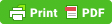# Physics Lacture series : Units and Measurement

Physical Quantities :- Any quantity which can be measure is called physical quantity. eg- length, mass, time, temperature, etc.
on the basis of units and measurement physical quantities can be divide in
• Fundamental quantities:- The physical quantities which do not depend on the other physical quantities are known as fundamental physical quantities. eg-  length, mass, electric current, time, temperature, etc.
• Derived quantities :-  All the physical quantities which are not the fundamental physical quantities but are derived from it  are known as derived physical quantities. eg- work, force, pressure, area, volume, etc.

on the basis of direction and magnitude physical quantity can be classified as
• Scalar quantities :-  A physical quantity which has only its magnitude but no direction is called physical quantity. eg-  distance, energy, power, time, speed, volume, density, etc
• Vector quantities :- A physical quantity which has magnitude and direction is called vector quantity. eg- displacement, velocity, momentum, force, etc.

Unit:-  The standard quantity used to measure the given physical quantity is called the unit.
eg - unit of time is second.
units are of two kinds :
• Fundamental units :-  A fundamental unit is that which is independent of any other unit or which can neither be changed nor can be related to any other fundamental unit. eg-  mass, length, time, temperature, current, etc.
• Derived units :-  Derived units are those which depend on the fundamental units are which can be expressed in the term of the fundamental units. eg-  area, volume, sports, etc.
• Systems of Units
A system of units is the complete set of units, both fundamental and derived, for all kinds of physical quantities. The common system of units which is used in mechanics are given below:
• CGS System In this system, the unit of length is centimetre, the unit of mass is gram and the unit of time is second.
• FPS System In this system, the unit of length is foot, the unit of mass is pound and the unit of time is second.
• MKS System In this system, the unit of length is metre, the unit of mass is kilogram and the unit of time is second.
• SI System This system contain seven fundamental units and two supplementary fundamental units.
• Some Physical quantities  and their SI units :-
No.    Fundamental
Quantities
SI unit
1LengthMeter (m)
2MassKg
3TimeSecond (s)
4TempratureKelvin (K)
5Electric current    Ampere (A)
6Luminous
intensity
Candela (cd)
7Amount of
Intensity
Mole
Supplementary Fundamental Units
Radian and steradian are two supplementary fundamental units. It measures plane angle and solid angle respectively
No.     Supplementary
Fundamental quantities
Supplementry
Unit
Symbol
Dimensions
Dimensions of any physical quantity are those powers which are raised on fundamental units to express its unit. The expression which shows how and which of the base quantities represent the dimensions of a physical quantity, is called the dimensional formula.
Dimensional Formula of Some Physical Quantities
No.Physical
quantity
Dimensional
Formula
SI unit
1Area[L²]
2Volume[L³]
3Velocity[LT⁻¹]m/s
4Acceleraton[LT⁻²]|m/s²
5Force[MLT⁻²]Newton
6Work or
Energy
[ML²T⁻²]Joule
7Power[ML²T⁻³]J/s or watt
8Pressure or
Stress
[ML⁻¹T⁻²]Nm⁻²
9Impulse[MLT⁻¹]kgms⁻¹
10Density[ML⁻³]kgm⁻³
11Modulus of
Elasticity
[ML⁻¹T-2]Nm⁻²
12Surface
Tension
[MT⁻²]Nm⁻¹
13Gravitational
Constant
[M⁻¹L³T⁻²]Nm²/kg²
14Moment of
Inertia
[ML²]kgm²
15Angular
velocity